# Javascript Arrays

Javascript Arrays

At times, we might require to store multiple data in our program. One way to store this data is by creating an array. The thing is that we can create an array, and store multiple values in that (we can also store the values of different data types, as can be seen in the below example here).

To create arrays, we have to make use of the square brackets([ ]). Within the square brackets, we are going to write the Javascript Arrays elements, and each element would be separated by a comma.

## Javascript Arrays

Have a look at the below example, which demonstrates how to create an array, with some elements.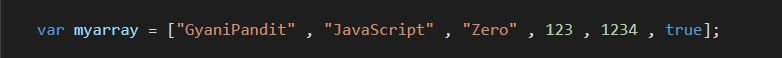When you have much data to store, creating variables for each data can be tedious work, but thanks to arrays, they solve the problem. You can save all the values under one variable and access them all with the help of an index.

So, now we are going to head up and see how can we access the elements from the array, because, we only have one variable over here, and there are so many elements in the array, so we need something, with the help of which, we can access the individual elements from the array, and that something is the index.

The array index starts from 0, which means that the first element in the array would be at the 0th position, and the last element would be at the n – 1 position, where n is the length of the array(more on this ahead, but length simply means the total number of elements in the array).

So, how are we going to access the array of elements? Well, the answer is so simple. Let’s say that we want to access the first element in the array, which is at index 0, so we just have to write the name of the variable, then we have to give the square brackets, and within the square brackets, we have to give the index of the element. Let’s have a look at the example below, which gives us a clearer idea about the same thing –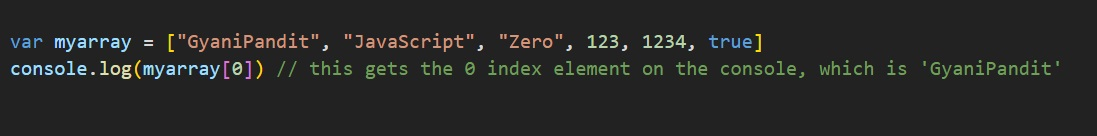As you can see, in the above program, we are trying to access the zero index element, which is the first element in the array. So, if you have a look at the output, the output comes out to be ‘GyaniPandit’.

So, if we want to access all the array elements one by one, we can just loop through the array, and access the elements according to the index. In order to do that, we should know the length of the array, because we need to know where we need to stop looping.

We are going to have a look at this later, how can we get the length of the array basically, which is very very easy?

Now, when we are able to access the particular array element with the help of an index, we can also change the element at that particular index. For example, in the above array, where it is true, we can just put something like ‘web development or anything.

Let’s have a look at how can we do it.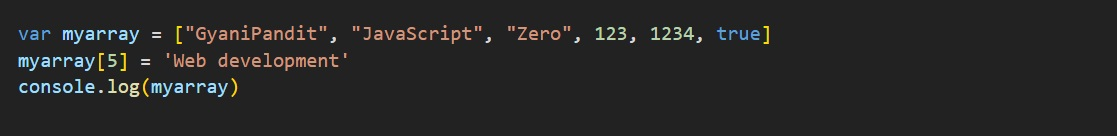In the above program, we can simply see that we are trying to access the 5th index element of the array, and just like we assign some value to some variable, we are assigning the value to the 5th index of the array. Also, we are printing the array afterward.

Let’s have a look at the output now –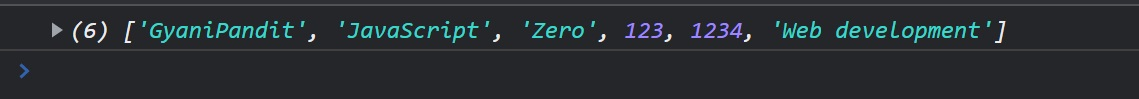As you can see from the output, the index 5 element has now changed to ‘Web development. So, whenever we are required to change the element at some index in the array, we can easily do it like how we did it above.

Let’s now have a program, which demonstrates all the things, like creating an array, accessing the elements from the Javascript Arrays, and changing/assigning values. Have a look –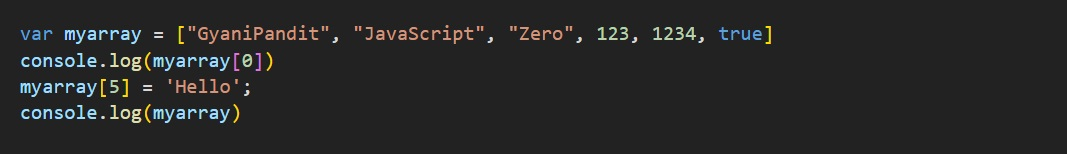In the above program, first of all, we have created an array, then we are trying to access the zero index element, and then we are assigning some new value to the index 5 from the array, and then we are printing the whole array. Have a look at the output now.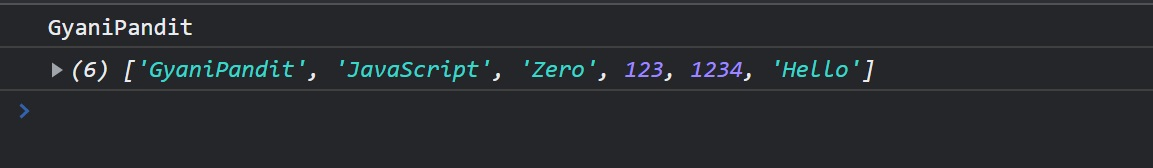So, from the above programs, we get an idea, about the different things, like creating an array, accessing the array elements, and assigning/changing the values at some given indexes.

Now, let’s turn to doing some different things, like getting the length of the array, and some other methods, which help us do different things, like pushing some element to the end of the array, or removing some element from the end of the array, and much more. But first of all, let’s have a look at how can we get the length of the given array.